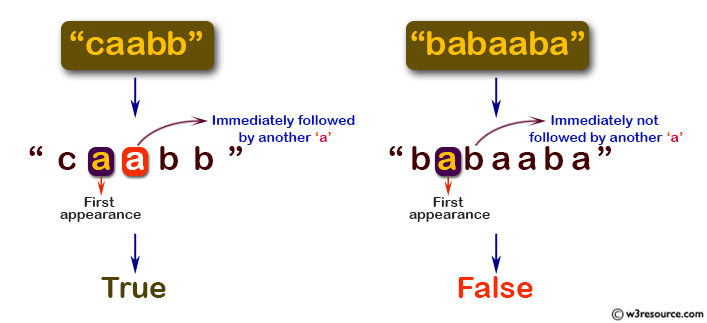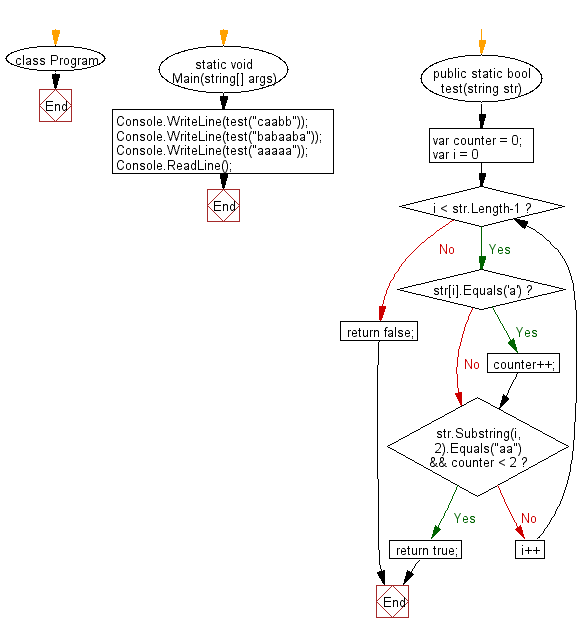﻿ C# - Check if a repeated a follows the first a in a string# C# Sharp Basic Algorithm Exercises: Check whether the first appearance of 'a' in a given string is immediately followed by another 'a'

## C# Sharp Basic Algorithm: Exercise-28 with Solution

Write a C# Sharp program to check whether the first appearance of "a" in a given string is immediately followed by another "a".

Pictorial Presentation:Sample Solution:-

C# Sharp Code:

``````using System;
namespace exercises
{
class Program
{
static void Main(string[] args)
{
Console.WriteLine(test("caabb"));
Console.WriteLine(test("babaaba"));
Console.WriteLine(test("aaaaa"));
}

public static bool test(string str)
{
var counter = 0;
for (var i = 0; i < str.Length-1; i++)
{
if (str[i].Equals('a')) counter++;
if(str.Substring(i, 2).Equals("aa") && counter < 2)
return true;
}
return false;
}
}
}
```
```

Sample Output:

```True
False
True```

Flowchart:C# Sharp Code Editor:

Improve this sample solution and post your code through Disqus

What is the difficulty level of this exercise?

Test your Programming skills with w3resource's quiz.

﻿